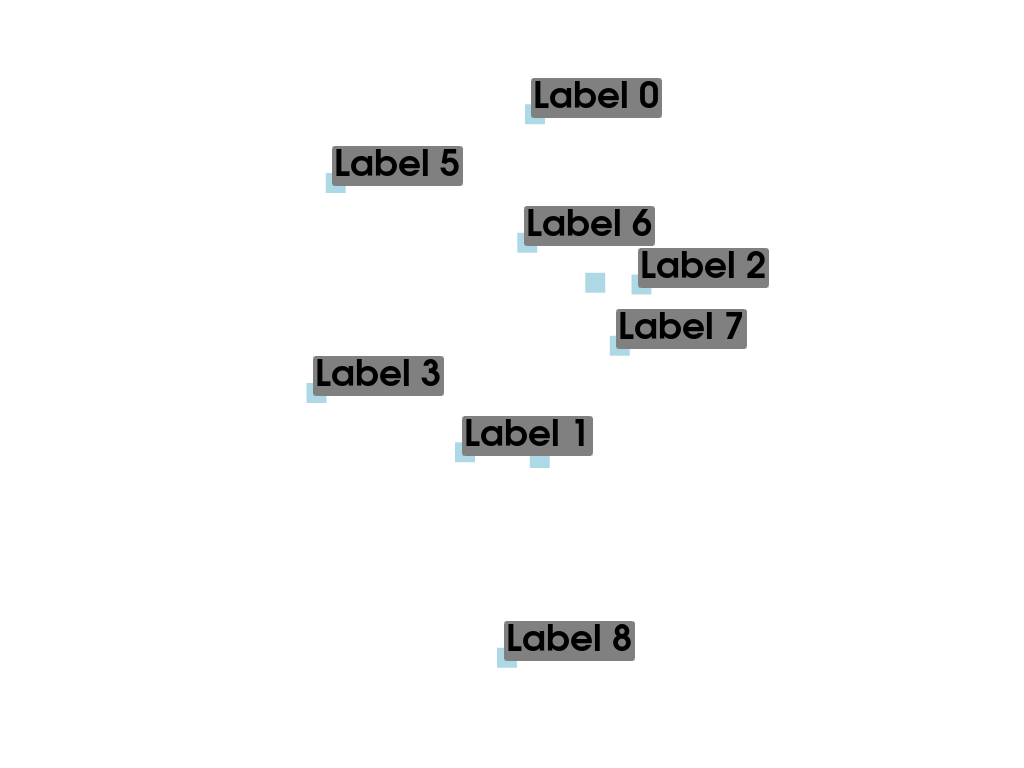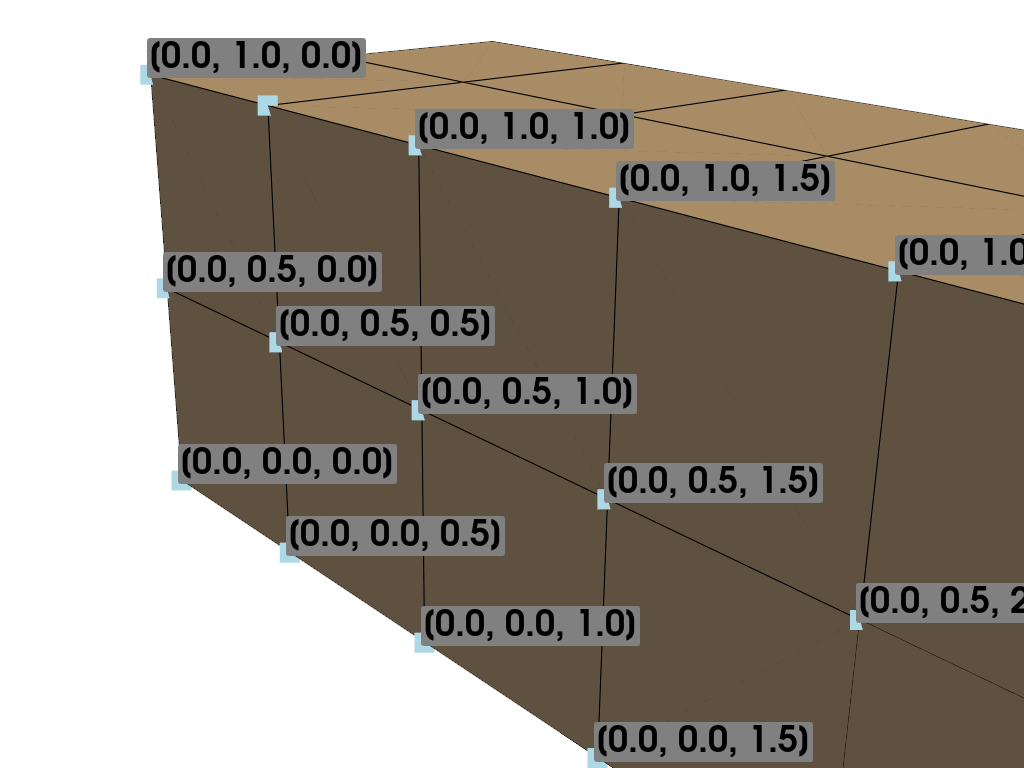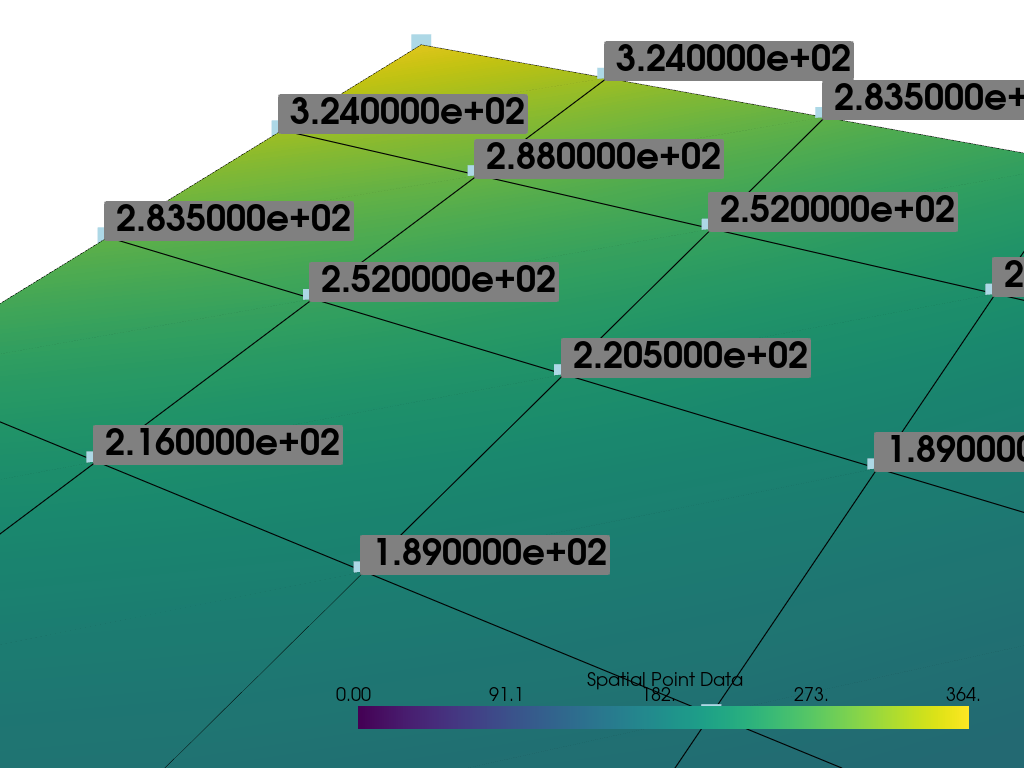# Label Points#

Use string arrays in a point set to label points

```import numpy as np
import pyvista as pv
from pyvista import examples
```

The `pyvista.Plotter.add_point_labels()` method makes it easy to add point labels to a scene.

```help(pv.Plotter.add_point_labels)
```
```Help on function add_point_labels in module pyvista.plotting.plotting:

add_point_labels(self, points, labels, italic=False, bold=True, font_size=None, text_color=None, font_family=None, shadow=False, show_points=True, point_color=None, point_size=None, name=None, shape_color='grey', shape='rounded_rect', fill_shape=True, margin=3, shape_opacity=1.0, pickable=False, render_points_as_spheres=False, tolerance=0.001, reset_camera=None, always_visible=False, render=True)
Create a point actor with one label from list labels assigned to each point.

Parameters
----------
points : sequence or pyvista.DataSet or vtk.vtkAlgorithm
An ``n x 3`` sequence points or pyvista dataset with points or
mesh-producing algorithm.

labels : list or str
List of labels.  Must be the same length as points. If a
string name is given with a :class:`pyvista.DataSet` input for
points, then these are fetched.

italic : bool, optional
Italicises title and bar labels.  Default ``False``.

bold : bool, optional
Bolds title and bar labels.  Default ``True``.

font_size : float, optional
Sets the size of the title font.  Defaults to 16.

text_color : ColorLike, optional
Color of text. Either a string, RGB sequence, or hex color string.

* ``text_color='white'``
* ``text_color='w'``
* ``text_color=[1.0, 1.0, 1.0]``
* ``text_color='#FFFFFF'``

font_family : str, optional
Font family.  Must be either ``'courier'``, ``'times'``,
or ``'arial``.

show_points : bool, optional
Controls if points are visible.  Default ``True``.

point_color : ColorLike, optional
Either a string, rgb list, or hex color string.  One of
the following.

* ``point_color='white'``
* ``point_color='w'``
* ``point_color=[1.0, 1.0, 1.0]``
* ``point_color='#FFFFFF'``

point_size : float, optional
Size of points if visible.

name : str, optional
The name for the added actor so that it can be easily
updated.  If an actor of this name already exists in the
rendering window, it will be replaced by the new actor.

shape_color : ColorLike, optional
Color of shape (if visible).  Either a string, rgb
sequence, or hex color string.

shape : str, optional
The string name of the shape to use. Options are ``'rect'`` or
``'rounded_rect'``. If you want no shape, pass ``None``.

fill_shape : bool, optional
Fill the shape with the ``shape_color``. Outlines if ``False``.

margin : int, optional
The size of the margin on the label background shape. Default is 3.

shape_opacity : float, optional
The opacity of the shape in the range of ``[0, 1]``.

pickable : bool, optional
Set whether this actor is pickable.

render_points_as_spheres : bool, optional
Render points as spheres rather than dots.

tolerance : float, optional
A tolerance to use to determine whether a point label is
visible.  A tolerance is usually required because the
conversion from world space to display space during
rendering introduces numerical round-off.

reset_camera : bool, optional
Reset the camera after adding the points to the scene.

always_visible : bool, optional
Skip adding the visibility filter. Default False.

render : bool, optional
Force a render when ``True`` (default).

Returns
-------
vtk.vtkActor2D
VTK label actor.  Can be used to change properties of the labels.

Examples
--------
>>> import numpy as np
>>> import pyvista
>>> pl = pyvista.Plotter()
>>> points = np.array([[0.0, 0.0, 0.0],
...                    [1.0, 1.0, 0.0],
...                    [2.0, 0.0, 0.0]])
>>> labels = ['Point A', 'Point B', 'Point C']
>>> actor = pl.add_point_labels(points, labels, italic=True, font_size=20,
...                             point_color='red', point_size=20,
...                             render_points_as_spheres=True,
>>> pl.camera_position = 'xy'
>>> pl.show()
```

## Label Point Cloud#

Let’s make a random point cloud and label each point in 3D space

```# Make some random points
poly = pv.PolyData(np.random.rand(10, 3))
```

Add string labels to the point data - this associates a label with every node:

```poly["My Labels"] = [f"Label {i}" for i in range(poly.n_points)]
poly
```
PolyDataInformation
N Cells10
N Points10
N Strips0
X Bounds4.404e-03, 6.464e-01
Y Bounds6.402e-02, 9.706e-01
Z Bounds9.953e-02, 9.460e-01
N Arrays1
NameFieldTypeN CompMinMax
My LabelsPoints1nannan

Now plot the points with labels using `pyvista.Plotter.add_point_labels()`

```# (your code here, answer below)
```
```plotter = pv.Plotter()
plotter.show()
```## Label Node Locations#

This example will label the nodes of a mesh with their coordinate locations

```# Load example beam file
grid = pv.UnstructuredGrid(examples.hexbeamfile)
```

Create plotting class and add the unstructured grid

```plotter = pv.Plotter()

# Add labels to points on the yz plane (where x == 0)
points = grid.points
mask = points[:, 0] == 0

plotter.camera_position = [(-1.5, 1.5, 3.0), (0.05, 0.6, 1.2), (0.2, 0.9, -0.25)]

plotter.show()
```## Label Scalar Values#

This example will label each point with their scalar values

```mesh = examples.load_uniform().slice()
```
```p = pv.Plotter()